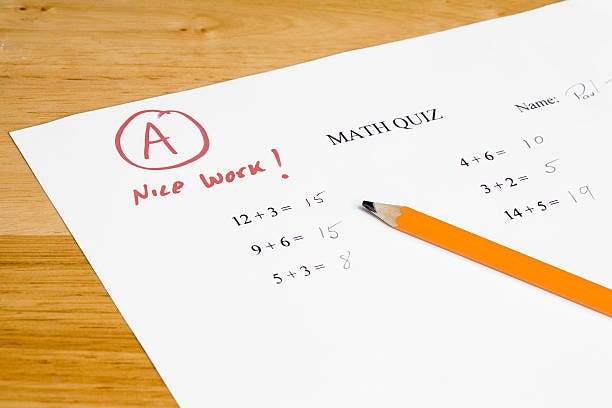Source: Deep Learning on Medium( Note: I have not added all the code here, if you want to check you can visit my GitHub where I have the complete tutorial in a ipynb notebook )

# WorKflow

There are two modules in the workflow Workspace detection module and Analysis Module. Workspace Detection module is responsible for detecting multiple work spaces in a given sheet of paper.

Analysis module is responsible for detecting and localizing characters in lines in any given single workspace, and mathematically analyzing them and then drawing red, green boxes depending upon their correctness.

# Workspace Detection

Workspace detection model assumes that there are valid rectangular boxes in the given scanned worksheet. This image below shows the worksheet design. Three rectangular boxes in this worksheet are the work-spaces.

## Step 1 : Finding Rectangular Boxes

Rectangles are formed by two horizontal and vertical lines. So the first step is to find all the valid rectangles ignoring digits, symbols or anything that is written on the worksheet.

This code below will first create a binary image called “vertical_lines_img” which contains the vertical lines that are present in the worksheet, then another binary image called “horizontal_lines_img” which contains horizontal lines that are present in the worksheet.